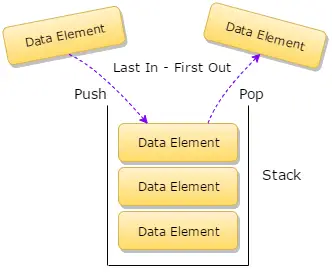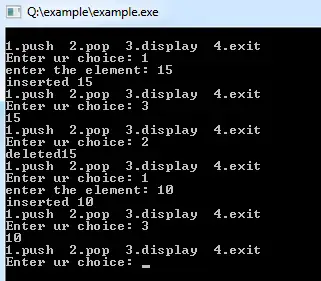# Concepts of Stack in Data Structure

In this chapter, you will explore one of the most important data structures which are used in many fields of programming and data handling, i.e., the Stack. It falls under the category of an abstract data type which serves as a concrete and valuable tool for problem-solving. In this chapter, you will study the various operations and working technique of stack data structure.

## What is stack?

A stack is a linear data structure in which all the insertion and deletion of data or you can say its values are done at one end only, rather than in the middle. Stacks can be implemented by using arrays of type linear.

The stack is mostly used in converting and evaluating expressions in Polish notations, i.e.:

• Infix
• Prefix
• Postfix

In case of arrays and linked lists, these two allows programmers to insert and delete elements from any place within the list, i.e., from the beginning or the end or even from the middle also. But in computer programming and development, there may arise some situations where insertion and deletion require only at one end wither at the beginning or end of the list. The stack is a linear data structure, and all the insertion and deletion of its values are done in the same end which is called the top of the stack. Let us suppose take the real-life example of a stack of plates or a pile of books etc. As the item in this form of data structure can be removed or added from the top only which means the last item to be added to the stack is the first item to be removed. So you can say that the stack follows the Last In First Out (LIFO) structure.

## Stack as Abstract Data Type

He stacks of elements of any particular type is a finite sequence of elements of that type together with the following operations:

• Initialize the stack to be empty
• Determine whether the stack is empty or not
• Check whether the stack is full or not
• If the stack is not full, add or insert a new node at the top of the stack. This operation is termed as Push Operation
• If the stack is not empty, then retrieve the node at its top
• If the stack is not empty, the delete the node at its top. This operation is called as Pop operation## Representation of Stack using Arrays

The stack can be represented in memory with the use of arrays. To do this job, you need to maintain a linear array STACK, a pointer variable top which contains the top element.

Example:

``````#include <iostream>
#include<stdlib.h>
using namespace std;

class stack {
int stk;
int top;

public:
stack()
{
top = -1;
}
void push(int x)
{
if (top >= 4) {
cout << "stack overflow";
return;
}
stk[++top] = x;
cout << "inserted " << x;
}
void pop()
{
if (top < 0) {
cout << "stack underflow";
return;
}
cout << "deleted " << stk[top--];
}
void display()
{
if (top < 0) {
cout << " stack empty"; return; } for (int i = top; i >= 0; i--)
cout << stk[i] << " ";
}
};

int main()
{
int ch;
stack st;
while (1) {
cout << "\n1.push 2.pop 3.display 4.exit\nEnter ur choice: "; cin >> ch;
switch (ch) {
case 1:
cout << "enter the element: "; cin >> ch;
st.push(ch);
break;
case 2:
st.pop();
break;
case 3:
st.display();
break;
case 4:
exit(0);
}
}
}``````

Output: